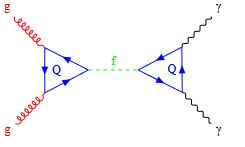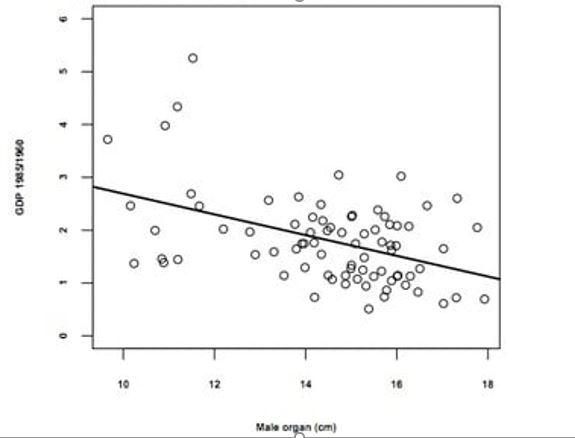# p-‘s and q-‘s reduxContinuing our saga, trying to be intellectually honest, while a little prurient (Look It Up!, to adopt a recent political slogan), let’s look at the ridiculous “measured” correlation in point 3 of this public post. Let’s call it the PPP-GDP correlation! The scatter graph with data is displayed belowDoes it make sense? As in all questions about statistics, a lot of the seminal work traces back to Fisher and Karl Pearson. The data in the graph can be (painfully) transcribed and the correlation computed in an  Excel spreadsheet. The result is a negative correlation – around -34% – higher GDP implies lower PPP. Sorry, men in rich countries. You don’t measure up!

Some definitions first. If you take two normally distributed random variables$X$ and$Y$ with means$\mu_X, \mu_Y$ and standard deviations$\sigma_X, \sigma_Y$, and you collect$N$ samples of pairs$(x_i, y_i)$,  then the Pearson coefficient$r = \frac{\sum_{i=1}^N(x_i - \mu_X^S)(y_i - \mu_Y^S)}{ \sqrt{\sum_{i=1}^N (x_i-\mu_X^S)^2} \sqrt{ \sum_{j=1}^N (y_j-\mu_Y^S)^2} }$

measures the correlation between the pairs of variables measured by considering this sample. If the sample were infinitely large, you would expect to recover the “actual” correlation$\rho$ between the two variables. They could then be assumed to be distributed through a joint (technical term “bivariate”) distribution for two random variables.

In general, this quantity$r$ has a rather complicated distribution. However, Fisher discovered that a certain variable (called the “Fisher” transform of r) is approximately normally distributed.$F(r) = \frac{1}{2} \ln \frac{1+r}{1-r} = arctanh(r)$

with mean$F(\rho)=arctanh(\rho)$ and standard deviation$\frac{1}{\sqrt{N-3}}$.

Once we know something is (at least approximately) normally distributed, we can throw all sorts of simple analytic machinery at it. For instance, we know that

• The probability that the variable is within 1.96 standard deviations of the mean (on either side) is 95%
• The probability that the variable is within 3 standard deviations of the mean (on either side) is 99.73%
• The probability that the variable is within 5 standard deviations of the mean (on either side) is 99.99994%

The$z-score$ is the number of standard deviations a sample is away from the mean of the distribution.

So, if we were sensible, we would start with a null hypothesis – there is no correlation between PPP and GDP.

If so, the expected correlation$\rho$ between these sets of variables, is 0.

The Fisher transform of$\rho=0$ is$F(0)=arctanh(0)=0$.

The standard deviation of the Fisher transform is$\frac{1}{\sqrt{N-3}}$. In the graph above,$N=75$, so the standard deviation is$0.11785$.

If you measured a correlation of$-0.34$ from the data, that corresponds to a Fisher transform of$-0.354$, which is a little more than 3 standard deviations away from the “expected” mean of 0!

If you went to the average Central banker with a 3 standard deviation calculation of what could go wrong (at least before the 2008 Financial crisis), he or she would have beamed at you in fond appreciation. Now, of course, we realize (as particle physicists have) that the world needs to be held to a much higher standard. In fact, if you hold to a 5 standard deviation bound, the correlation could be between -52.9% and +52.9%.

So, if you fondly held the belief expressed in graph 3 of the post alluded to above, you might need to think again.

If you had been following the saga of the 750 GeV “bump” discovered in the data from the Large Hadron Collider a few years ago, that was roughly at the 3.5 standard deviation level. If you held off from publishing a theory early describing exactly which particle had been observed, you would have been smart. The data, at that level, was as believable as the PPP vs GDP data above. The puzzling thing, in my opinion, is why the “independent” detectors ATLAS and CMS saw the same sort of bump in the same places. Speaks to a level of cross-talk which is not supposed to happen!

The above calculation leads to a very simple extension of the p-value concept to correlation. It’s just the probability of seeing correlations more extreme than the one observed, given the null hypothesis. The choice of the null hypothesis doesn’t necessarily have be a correlation of$0$. It might be reasonable to expect, for instance in the case of the correlation between the Japanese equity index and the exchange rate between the Yen and the dollar, that there is some stable (non-zero) correlation over at least one business cycle.

Featured image courtesy Maximilian Reininghaus

Buzzfeed post by Ky Harlin (Director of Data Science, Buzz Feed)

I haven’t bothered, in this analysis, to consider how the data was collected and whether it is even believable. We probably have a lot of faith in how the GDP increase data was computed, though methods have obviously changed in the last sixty years. However, did they use cadavers, to measure “lengths”?  Did they wander around poor villages with tape measures? How many of the data collectors survived the experience? This graph has all the signs of being an undergraduate alcohol-fueled experiment with all the attendant bias risks.

If you liked this post, you might like this website.